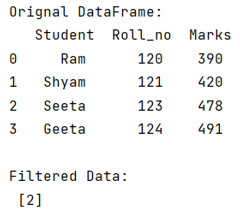# How to return the index of filtered values in pandas DataFrame?

Given a Pandas DataFrame, we have to return the index of filtered values.
Submitted by Pranit Sharma, on June 23, 2022

Pandas is a special tool that allows us to perform complex manipulations of data effectively and efficiently. Inside pandas, we mostly deal with a dataset in the form of DataFrame. DataFrames are 2-dimensional data structures in pandas. DataFrames consist of rows, columns, and the data.

## Return the index of filtered values in pandas DataFrame

To return the index of filtered values in pandas DataFrame, we will first filter the column values by comparing them against a specific condition, then we will use the index() method to return the index of the filtered value. We can also store all the filtered values into a list by using the tolist() method.

Note:

To work with pandas, we need to import pandas package first, below is the syntax:

```import pandas as pd
```

Let us understand with the help of an example,

## Python program to return the index of filtered values in pandas DataFrame

```# Importing pandas package
import pandas as pd

# Creating a dictionary
d= {
'Student':['Ram','Shyam','Seeta','Geeta'],
'Roll_no':[120,121,123,124],
'Marks':[390,420,478,491]
}

# Create a DataFrame
df = pd.DataFrame(d)

# Display original DataFrame
print("Orignal DataFrame:\n",df,"\n")

# Filter values
result = df[df['Student'] == 'Seeta'].index.tolist()

# Display result
print("Filtered Data:\n",result)
```

## Output

The output of the above program is: